# Surface Tension.ppt

designer at bijender jitender & co um bijender jitender & co
26. Jul 2022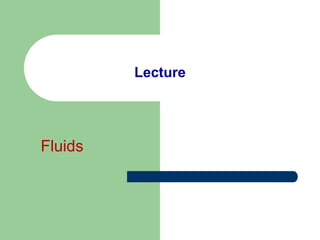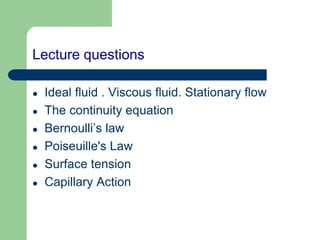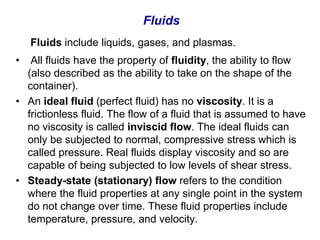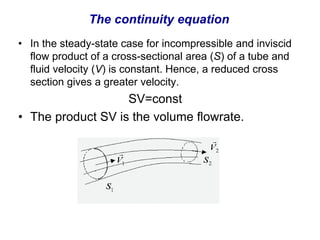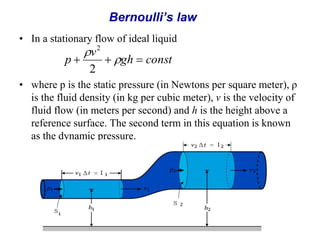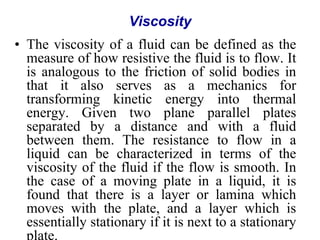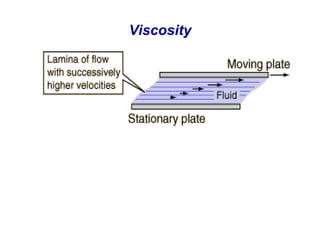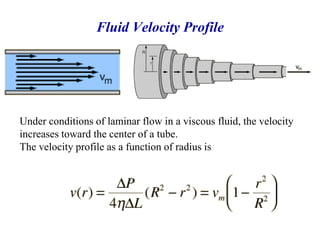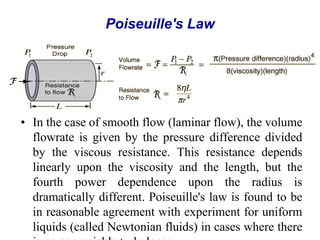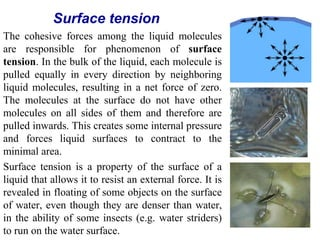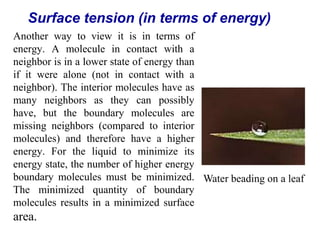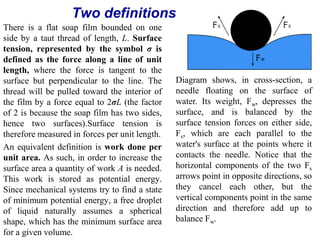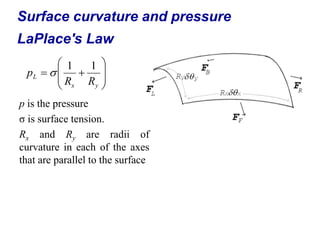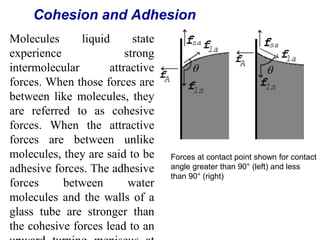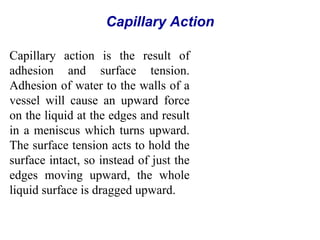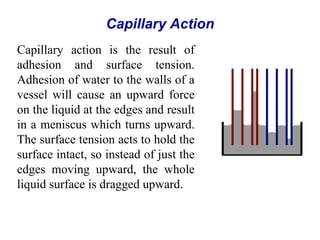1 von 17

### Surface Tension.ppt

• 2. Lecture questions  Ideal fluid . Viscous fluid. Stationary flow  The continuity equation  Bernoulli’s law  Poiseuille's Law  Surface tension  Capillary Action
• 3. Fluids Fluids include liquids, gases, and plasmas. • All fluids have the property of fluidity, the ability to flow (also described as the ability to take on the shape of the container). • An ideal fluid (perfect fluid) has no viscosity. It is a frictionless fluid. The flow of a fluid that is assumed to have no viscosity is called inviscid flow. The ideal fluids can only be subjected to normal, compressive stress which is called pressure. Real fluids display viscosity and so are capable of being subjected to low levels of shear stress. • Steady-state (stationary) flow refers to the condition where the fluid properties at any single point in the system do not change over time. These fluid properties include temperature, pressure, and velocity.
• 4. The continuity equation • In the steady-state case for incompressible and inviscid flow product of a cross-sectional area (S) of a tube and fluid velocity (V) is constant. Hence, a reduced cross section gives a greater velocity. SV=const • The product SV is the volume flowrate.
• 5. Bernoulli’s law • In a stationary flow of ideal liquid • where p is the static pressure (in Newtons per square meter), ρ is the fluid density (in kg per cubic meter), v is the velocity of fluid flow (in meters per second) and h is the height above a reference surface. The second term in this equation is known as the dynamic pressure. const gh v p      2 2
• 6. Viscosity • The viscosity of a fluid can be defined as the measure of how resistive the fluid is to flow. It is analogous to the friction of solid bodies in that it also serves as a mechanics for transforming kinetic energy into thermal energy. Given two plane parallel plates separated by a distance and with a fluid between them. The resistance to flow in a liquid can be characterized in terms of the viscosity of the fluid if the flow is smooth. In the case of a moving plate in a liquid, it is found that there is a layer or lamina which moves with the plate, and a layer which is essentially stationary if it is next to a stationary plate.
• 8. Fluid Velocity Profile Under conditions of laminar flow in a viscous fluid, the velocity increases toward the center of a tube. The velocity profile as a function of radius is
• 9. Poiseuille's Law • In the case of smooth flow (laminar flow), the volume flowrate is given by the pressure difference divided by the viscous resistance. This resistance depends linearly upon the viscosity and the length, but the fourth power dependence upon the radius is dramatically different. Poiseuille's law is found to be in reasonable agreement with experiment for uniform liquids (called Newtonian fluids) in cases where there
• 10. Surface tension The cohesive forces among the liquid molecules are responsible for phenomenon of surface tension. In the bulk of the liquid, each molecule is pulled equally in every direction by neighboring liquid molecules, resulting in a net force of zero. The molecules at the surface do not have other molecules on all sides of them and therefore are pulled inwards. This creates some internal pressure and forces liquid surfaces to contract to the minimal area. Surface tension is a property of the surface of a liquid that allows it to resist an external force. It is revealed in floating of some objects on the surface of water, even though they are denser than water, in the ability of some insects (e.g. water striders) to run on the water surface.
• 11. Surface tension (in terms of energy) Another way to view it is in terms of energy. A molecule in contact with a neighbor is in a lower state of energy than if it were alone (not in contact with a neighbor). The interior molecules have as many neighbors as they can possibly have, but the boundary molecules are missing neighbors (compared to interior molecules) and therefore have a higher energy. For the liquid to minimize its energy state, the number of higher energy boundary molecules must be minimized. The minimized quantity of boundary molecules results in a minimized surface area. Water beading on a leaf
• 12. Two definitions There is a flat soap film bounded on one side by a taut thread of length, L. Surface tension, represented by the symbol σ is defined as the force along a line of unit length, where the force is tangent to the surface but perpendicular to the line. The thread will be pulled toward the interior of the film by a force equal to 2σL (the factor of 2 is because the soap film has two sides, hence two surfaces).Surface tension is therefore measured in forces per unit length. An equivalent definition is work done per unit area. As such, in order to increase the surface area a quantity of work A is needed. This work is stored as potential energy. Since mechanical systems try to find a state of minimum potential energy, a free droplet of liquid naturally assumes a spherical shape, which has the minimum surface area for a given volume. Diagram shows, in cross-section, a needle floating on the surface of water. Its weight, Fw, depresses the surface, and is balanced by the surface tension forces on either side, Fs, which are each parallel to the water's surface at the points where it contacts the needle. Notice that the horizontal components of the two Fs arrows point in opposite directions, so they cancel each other, but the vertical components point in the same direction and therefore add up to balance Fw.
• 13. Surface curvature and pressure LaPlace's Law p is the pressure σ is surface tension. Rx and Ry are radii of curvature in each of the axes that are parallel to the surface           y x L R R p 1 1 
• 14. Cohesion and Adhesion Molecules liquid state experience strong intermolecular attractive forces. When those forces are between like molecules, they are referred to as cohesive forces. When the attractive forces are between unlike molecules, they are said to be adhesive forces. The adhesive forces between water molecules and the walls of a glass tube are stronger than the cohesive forces lead to an Forces at contact point shown for contact angle greater than 90° (left) and less than 90° (right)
• 15. Capillary Action Capillary action is the result of adhesion and surface tension. Adhesion of water to the walls of a vessel will cause an upward force on the liquid at the edges and result in a meniscus which turns upward. The surface tension acts to hold the surface intact, so instead of just the edges moving upward, the whole liquid surface is dragged upward.
• 16. Capillary Action Capillary action is the result of adhesion and surface tension. Adhesion of water to the walls of a vessel will cause an upward force on the liquid at the edges and result in a meniscus which turns upward. The surface tension acts to hold the surface intact, so instead of just the edges moving upward, the whole liquid surface is dragged upward.
• 17. Thank you for your attention !